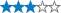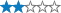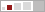<

## AGTSSP - Stage 6 - Hide and seek

A cache by parmstro Message this owner
Hidden : 03/08/2010
Difficulty:Terrain:Size:(small)

#### Watch

How Geocaching WorksPlease note Use of geocaching.com services is subject to the terms and conditions in our disclaimer.

### Geocache Description:

GEOCACHING ON NATIONAL TRUST PROPERTY IS AT YOUR OWN RISK.

Also, please note that the National Trust is caring for the specialist habitats and landscape you enjoy on this and other caches on NT property, which is one of the reasons we are glad to be members.

Stage 6 - a standard puzzle

I have used the common abbreviations for a couple, and a common alternative for another. 10=0 as usual.

 S A D E X P L O R A T I O N K P N L Y L L O S R D B A S H F U L E B C A C H E U N U T T E R C R K O R E D I R X E L A Q P T F E C Y E N O H Y R A G R L N J S K I V Y M I L L W W A E A H A A L H C T O C O E G N P M E A P T A C T Z O F T F G I S R O S U S W G E D T N E E N T I G G D K A T N L O N D L E E N K J L N A F A I G R A P A L D C T C O A I S O R G I I P T O A D N T S L A A C P A C P R O P M H L F S L F N T S K L A R O A M I N T O U A A A H E M S G G I T H O O P G P N L Y R A E W D N A E R O S T O O F

....Here is a link to the hints pages, which will help you to solve the puzzle, and which you can click on if you get stuck.

Lbh fubhyq raq hc jvgu 10 yrggref.
Cvfn - va natyr

Decryption Key

A|B|C|D|E|F|G|H|I|J|K|L|M
-------------------------
N|O|P|Q|R|S|T|U|V|W|X|Y|Z

(letter above equals below, and vice versa)